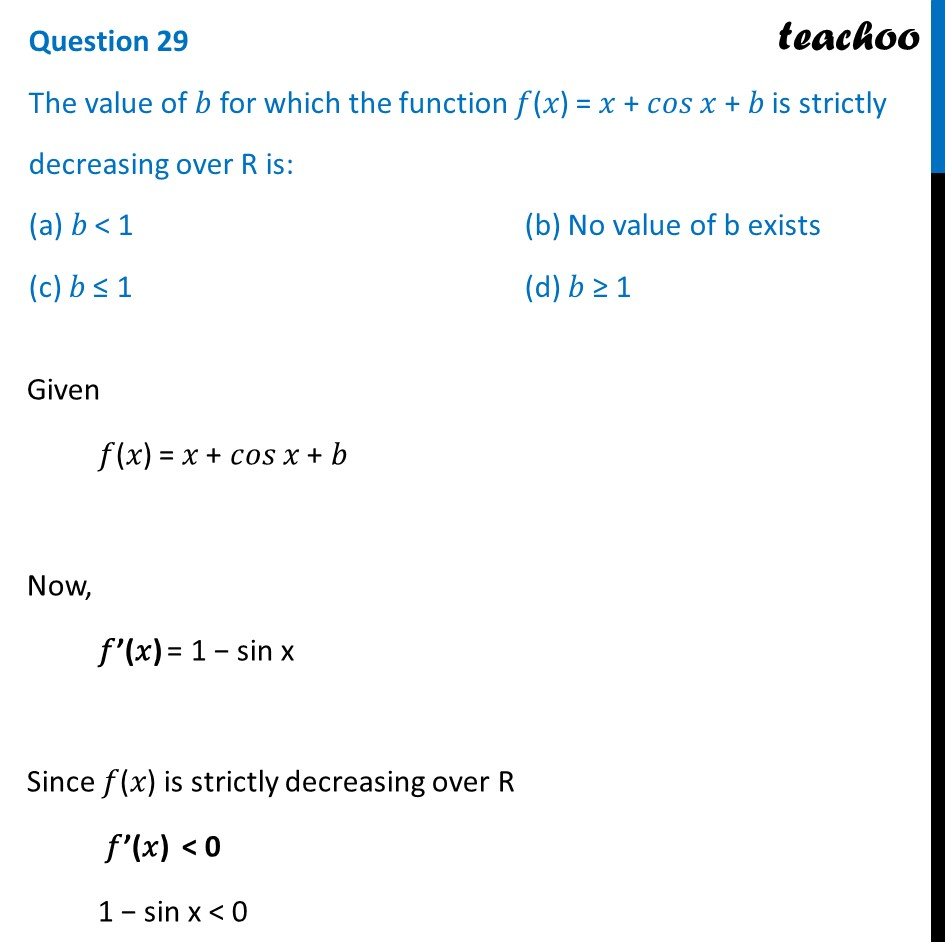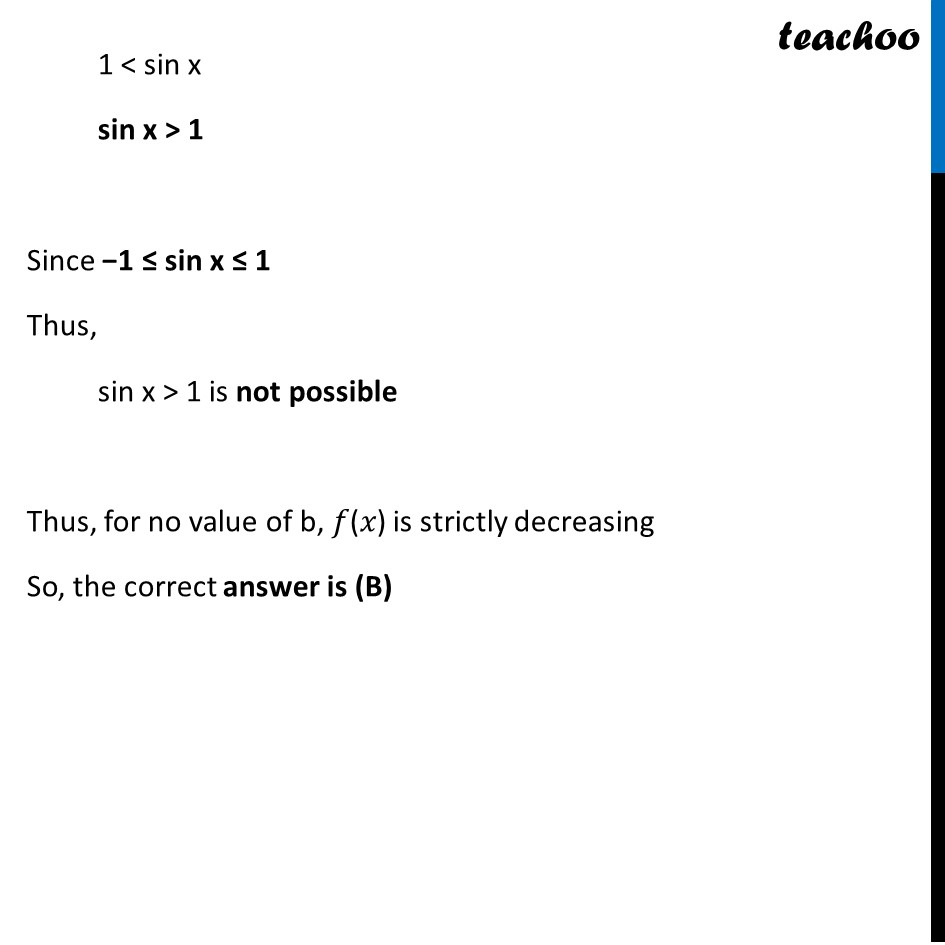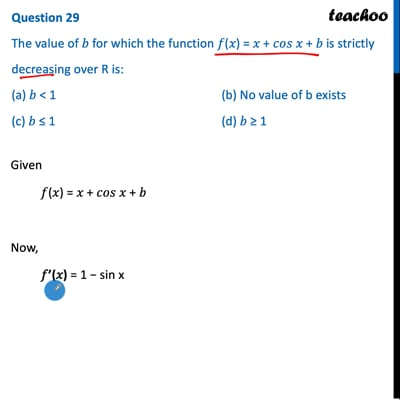CBSE Class 12 Sample Paper for 2022 Boards (MCQ Based - for Term 1)

Class 12
Solutions of Sample Papers and Past Year Papers - for Class 12 Boards

## (c) 𝑏 ≤ 1  (d) 𝑏 ≥ 1This video is only available for Teachoo black users

Introducing your new favourite teacher - Teachoo Black, at only ₹83 per month

### Transcript

Question 29 The value of 𝑏 for which the function 𝑓(𝑥) = 𝑥 + 𝑐𝑜𝑠 𝑥 + 𝑏 is strictly decreasing over R is: (a) 𝑏 < 1 (b) No value of b exists (c) 𝑏 ≤ 1 (d) 𝑏 ≥ 1 Given 𝑓(𝑥) = 𝑥 + 𝑐𝑜𝑠 𝑥 + 𝑏 Now, 𝑓’(𝑥) = 1 − sin x Since 𝑓(𝑥) is strictly decreasing over R 𝑓’(𝑥) < 0 1 − sin x < 0 1 < sin x sin x > 1 Since −1 ≤ sin x ≤ 1 Thus, sin x > 1 is not possible Thus, for no value of b, 𝑓(𝑥) is strictly decreasing So, the correct answer is (B)